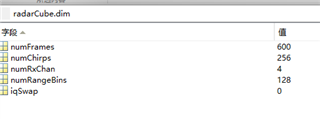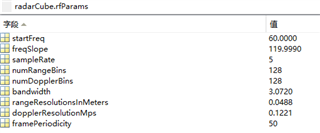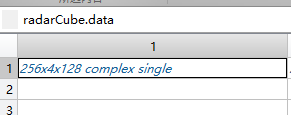#### 由于国庆节假期，帖子审批以及工程师回复将会有所延迟，敬请谅解

This thread has been locked.

If you have a related question, please click the "Ask a related question" button in the top right corner. The newly created question will be automatically linked to this question.

# DCA1000EVM: IWR6843AOPEVM + ICBOOST+DCA1000 通过mmwavestudio设置采集帧数较大时，原始数据处理问题

Part Number: DCA1000EVM
Other Parts Discussed in Thread: IWR6843AOPchannel1_data = test_data(10,1,:);
channel2_data = test_data(10,2,:);
channel3_data = test_data(10,3,:);
channel4_data = test_data(10,4,:);

channel1 = reshape(channel1_data,1,128);
channel2 = reshape(channel2_data,1,128);
channel3 = reshape(channel3_data,1,128);
channel4 = reshape(channel4_data,1,128);

N = 128;      %N-FFT

fft_channel1 = fft(channel1,N)./N;
fft_channel2 = fft(channel2,N)./N;
fft_channel3 = fft(channel3,N)./N;
fft_channel4 = fft(channel4,N)./N;

abs_channel1 = abs(fft_channel1);
abs_channel2 = abs(fft_channel2);
abs_channel3 = abs(fft_channel3);
abs_channel4 = abs(fft_channel4);

figure;
plot(abs_channel1);

figure;
plot(abs_channel2);

figure;
plot(abs_channel3);

figure;
plot(abs_channel4);图1图2                                                                                                                    图3图4

• 你好，